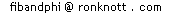# The Golden String of 0s and 1s

Other names for the Rabbit Sequence are the Golden Sequence because, as we shall see, it is closely related to the golden section numbers Phi (=1·6180339..) and phi=(0·6180339..).

## Contents

The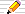icon means there is a Things to do investigation at the end of the section.# Fibonacci Numbers and the Rabbit sequence

This page is all about a remarkable sequence of 0s and 1s which is intimately related to the Fibonacci numbers and to Phi:
1 0 1 1 0 1 0 1 1 0 1 1 0 1 0 1 1 0 1 0 1 1 0 1 1 0 1 0 1 1 0 1 ...

First we re-examine Fibonacci's original Rabbit problem and see how it can generate an infinite sequence of two symbols and in a later section we see how the same sequence is very simply related to Phi also.

## Lining up the Rabbits

If we return to Fibonacci's original problem - about the rabbits (see the Fibonacci home page if you want to remind yourself) then we start with a single New pair of rabbits in the field. Call this pair N for "new".
```  Month 0:
N
```

Next month, the pair become Mature, denoted by "M".

```  Month 0:  1:
N   M
```

The following month, the M becomes "MN" since they have produced a new pair (and the original pair also survives).

```  Month 0:  1:  2:
N   M   M
N
```

The M of month 2 become MN again and the N of month 2 has become M, so month 3 is: "MNM"

```  Month 0:   1:   2:   3:
N -  M -  M -  M
\   \  N
N -  M
```

The next month it is "MNMMN".

The general rule is

replacing every M in one month by MN in the next and similarly replace every N by M.
Hence MNM goes to MN M MN .

We have now got a collection of sequences of M's and N's which begins: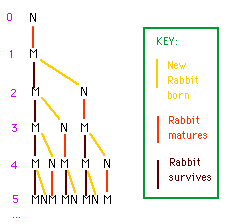```   0: N            =N

1: M            =M

2: M      N     =MN

3: M   N  M     =MNM

4: M N M  M N   =MNMMN

5: MNM MN MNM   =MNMMNMNM
...           ...
```

Compare this with the picture we had of the Rabbit Family Tree where sometimes M is replaced by NM and sometimes by MN.

We often use 1s and 0s for this sequence, so here we have replaced M by 1 and N by 0:

## Another way to generate The Rabbit sequence

We can make the rabbit sequence for month x by taking the sequence from month x-1 and writing it out again, following it by a copy of the sequence of month x-2.
So, starting from N and M the next is M (last month) followed by N (the previous month) giving MN.
The next will be MN followed by M = MNM
and the one after that is MNM followed by MN = MNMMN.

From this definition we can see that
each monthly sequence is the start of the following month's sequence.

This means that (after the first sequence which begins with N), there is really just one infinitely long sequence, which we call the rabbit sequence or the golden sequence or the golden string.

## Computers use The Rabbit sequence!

In this section we show how the definition of the Fibonacci numbers leads us directly to the Fibonacci Rabbit sequence, but this time we use 0s and 1s instead of Ms and Ns.
We see how a computer actually carries out the evaluation of a Fibonacci number using the Rabbit sequence secretly behind the scenes!

We can write a computer program to compute the Fibonacci numbers using the recursive definition:

f(0)=0
f(1)=1
f(n)=f(n-1)+f(n-2) for n>1
We will be interested in how the computer is evaluating a call of f on a number n - in particular, what are the actual numbers added (and in what order) when computing f(n). The third line of the definition means that to compute f(n) we first need to compute f(n-1) as a separate computation and then remember its result so that, when we have then computed f(n-2) - another separate computation - we can add the two values to find f(n). The first line of the definition means that
to compute f(0)
the program function immediately returns the answer 0.
The second line of the definition means that
to compute f(1)
the computer again immediately returns the answer 1.

We will examine the calls to the function f and represent them in diagrams of "calling sequences" so that we have the following diagram for f(0):

```f(0)
0
```
to show that
a call of f(0) is replaced by (gets expanded to) 0

Similarly,
```f(1)
1
```
shows that f(1) gets expanded to 1, shown on the line below it, using the function definition given above.
What happens for larger values of n?
To compute f(2)
since n>1 we will be using the third line of the definition
f(n)=f(n-1)+f(n-2)
For f(2), n is 2 so we need to compute f(1)+f(0).
First f(1) is computed, giving 1 and then we compute and add on f(0), which is recomputed as 0. The pattern of calls of f when computing f(2) is therefore shown in our calling sequence diagram as follows:
```f(2)
f(1)+f(0)
1    0
```

To compute f(3)
the function tells us to call f(2) and f(1) to compute f(2)+f(1). f(2) is called first, repeating the above computations and eventually returning 1+0=1 and after this f(1) is called, returning 1, so the final result of (1+0)+1=2 is returned.
In this case, the calling sequence in the computer again forms a "tree":

```f(3)
f(2)......+f(1)
f(1)+f(0)    1
1    0
```
Note that the actual additions performed are 1+0+1, and that these numbers appear the lower end of the "branches" in the "calling tree".
A note on trees in computing
In computing science such tree diagrams are very useful and they appear in many different situations.
The natural way to represent them is as above, where the "root" from which the "tree" grows is at the top (since we read from top down a page of text) and so the ends of the "branches" - often called "leaves" - appear at the lowest level! So our trees are antipodean i.e Australian since they grow upside-down!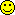For f(4)
the calling sequence tree is f(3) as in the last calling tree diagram but now including the call of f(2) since f(4)=f(3)+f(2):

```f(4)
f(3)............+f(2)
f(2)......+f(1)  f(1)+f(0)
f(1)+f(0)    1     1    0
1    0
```
so the actual addition performed is
1+0+1+1+0

If we consider further calls of f(n) for n=5 and above
then since f(n)=f(n-1)+f(n-2), each tree begins with the previous tree [used to compute f(n-1)] and is followed by the whole of the tree before that, namely for f(n-2).

For instance, here is the calling tree for f(5) which starts with f(4) and, on the right, we include f(3):

```f(5)
f(4).......................+f(3)
f(3)............+f(2)       f(2)......+f(1)
f(2)......+f(1)  f(1)+f(0)  f(1)+f(0)    1
f(1)+f(0)    1     1    0     1    0
1    0
```
The actual additions this time are
1+0+1+1+0+1+0+1=5
You should now be able to see that the sequence of 0's and 1's used in the additions is defined as follows: let's let s(n) stand for the sequence of 0's and 1's used in computing f(n) so that:
s(0)=0
s(1)=1
s(n)=s(n-1)
"followed by" s(n-2)
so we have
```                                    number of
0s  1s in s(n):
s(0)=0                          =0  1   0
s(1)=1                          =1  0   1
s(2)=1+0                        =1  1   1
s(3)=1+0+1                      =2  1   2
s(4)=1+0+1+1+0                  =3  2   3
s(5)=1+0+1+1+0+1+0+1            =5  3   5
s(6)=1+0+1+1+0+1+0+1+1+0+1+1+0  =8  5   8
...
```
and we see s(n) gives a sequence of additions involving 0s and 1s which defined the Fibonacci numbers.

There is no "last" sequence in the s(n) series but we see that a unique sequence of infinitely many 0's and 1's is defined by this process and is the one we call the the Fibonacci Rabbit sequence or the Golden Sequence.

### The number of additions when computing f(n)

When computing f(n) by the recursive formula at the start of this section:
f(0)=0; f(1)=1; f(n)=f(n-1)+f(n-2) for n<0 or n>1
it takes longer to compute the larger values. This is because the computer is doing a lot of recalculation as we have just seen above. So we can ask
How much work does it take to compute f(n)?
This is measured by the number of additions performed.
We have already written out the actual additions in the table above, up to s(6). Let's look at it again and count the number of addition operations this time:
```                                    number of +'s
s(0)=0                          0
s(1)=1                          0
s(2)=1+0                        1
s(3)=1+0+1                      2
s(4)=1+0+1+1+0                  4
s(5)=1+0+1+1+0+1+0+1            7
s(6)=1+0+1+1+0+1+0+1+1+0+1+1+0  12
...
```
What is the pattern in the series 0,0,1,2,4,7,12,...?
Let's call this the A series (for Additions):
 n: A(n): 0 1 2 3 4 5 6 .. 0 0 1 2 4 7 12 ..
We can see some information by just looking at the recursion formula:
f(n) = f(n-1)+ f(n-2)
so
A(n) is the number of additions in computing F(n-1)
PLUS the number of additions in computing F(n-2)
PLUS 1 in order to add f(n-1) to f(n-2)
or, using the A(i) notation for 'the number of additions in computing f(i)':
A(n) = A(n-1) + A(n-2) + 1; A(0)=0; A(1)=0
This is now a complete (recursive) definition of A. We can now use it to find A(7), the number of additions needed to compute f(7) (=13).
It is A(6)+A(5)+1 or 7+12+1 which is 20.
Here are a few more values:
 n: A(n): 0 1 2 3 4 5 6 7 8 9 10 0 0 1 2 4 7 12 20 33 54 88

There is another of the Fibonacci surprises here. Though the numbers are not the Fibonacci numbers, they have a similar method of construction (add the last two and then add 1). Have you noticed how the A series is related to the Fibonacci numbers themselves? The answer....

The A numbers are just 1 less than a Fibonacci number:

 n: A(n): f(n+1): 0 1 2 3 4 5 6 7 8 9 10 0 0 1 2 4 7 12 20 33 54 88 1 1 2 3 5 8 13 21 34 55 89
So
A(n) = f(n+1) - 1
This means that the work needed to compute f(n) is measured by f(n+1) because we can ignore the 'minus 1' as it is insignificant when f(n) is large.

With thanks to Aaron Goh for suggesting this section.

# Phi and the Rabbit sequence

Our "golden" sequence has many remarkable properties that involve the golden section.

## The Phi line Graph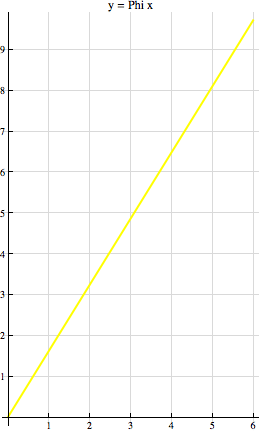If we draw the line y = Phi x on a graph, (ie a line whose gradient is Phi) then we can see the Rabbit sequence directly.

Where the Phi line crosses a horizontal grid line (y=1, y=2, etc) we write 1 by it on the line and where the Phi line crosses a vertical grid line (x=1, x=2, etc) we record a 0.

Now as we travel along the Phi line from the origin, we meet a sequence of 1s and 0s - the Rabbit sequence again!

The following sections explore this relationship using functions such as "the next integer below" (the floor function) and "the next integer above" (the ceiling function) which will tell us which grid-line we have just crossed.

## The rabbit sequence defined using the whole part of Phi multiples

If we take the number Phi, which we have seen is closely related to the Fibonacci series, then it leads to another simple definition of the rabbit sequence.
With the definitions above, we have to find all the preceding bits (Ms or Ns) to find which letter occurs in place i in the sequence. Using Phi=1·618034... we can compute it directly:
If we let M = 1 and N=0 then the rabbit sequence is 101101... and:
```   rabbit(i)=trunc((i+1)*Phi)-trunc(i*Phi) -1  OR
rabbit(i)=trunc((i+1)*phi)-trunc(i*phi)
where Phi=(sqrt(5)+1)/2=1·618034... and phi=Phi-1=(sqrt(5)-1)/2=0·618034...
```
"Trunc(x)" is the function which just forgets anything after a decimal point in x, for both positive and negative x.
To see how this works, look at this table:
```   i     i*Phi      trunc(i*Phi) diff diff-1 RabSeq
1     1.618034..    1
2     1   M
2     3.223606..    3
1     0   N
3     4.854101..    4
2     1   M
4     6.472135..    6
2     1   M
5     8.090169..    8
1     0   N
6     9.708203..    9
2     1   M
7    11.326237..   11     ...
```
where diff is the difference between the trunc item of the row above and the row following with 1=M and 0=N.

####Things to do1. Try extending the table for a few more rows.
2. Use phi=Phi-1 instead of Phi in the table but don't subtract 1 from the diffs.

## The rabbit sequence defined using the fractional parts of Phi multiples

Here is another method to generate the Rabbit sequence but this time using the bits we threw away above - the fractional parts of the multiples of Phi!
```   i     i*Phi      frac(i*Phi)    R or L?
1     1·618034..    0·618034..
2     3·223606..    0·223606..    L
3     4·854101..    0·854101..    R
4     6·472135..    0·472135..    L
5     8·090169..    0·090169..    L
6     9·708203..    0·708203..    R
7    11·326237..    0·326237..    L
...
"R or L?" means that the fractional part on that line=frac(i*Phi)
is moRe or Less than the fractional value on the line above=frac((i-1)*Phi)
```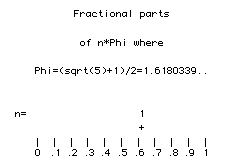An alternative way to generate the sequence of R and L is to look at this Quicktime movie of the fractional parts of the first 56 multiples of Phi (click on the picture).

Does the point move to the Right of the previous one or to the Left.

(Tip: Use the slider on the Quicktime movie frame to advance the picture one frame at a time.)

####Things to do1. Note that sometimes a new point will be plotted further to the right than any previous one (i.e. its fractional part will be larger than any before it). What multiples of Phi result in these "furthest out" points?
2. What multiples correspond to those points plotted furthest to the left?

## The rabbit sequence and the "spectrum" of Phi

Let's look again at the multiples of Phi, but this time concentrating on the whole number part of the multiples of Phi. We will find another extraordinary relationship.
The "whole number part" of x is written as floor(x) so we are looking at floor(i Phi) for i=1,2,3,.. .
In this section on the Golden String (the Rabbit sequence) will only be interested in positive numbers, so the floor function is the same as the trunc function.
 The sequence of truncated multiples of a real number R is called the spectrum of R.

Here are the first few numbers of the spectrum of Phi, that is the values of the series floor(Phi), floor(2 Phi), floor(3 Phi), floor(4 Phi), ..:-

 i i Phi trunc(i*Phi) 1 2 3 4 5 6 7 8 ... 1.618 3.236 4.854 6.472 8.09 9.708 11.326 12.944 ... 1 3 4 6 8 9 11 12 ...
So the spectrum of Phi is the infinite series of numbers beginning 1, 3, 4, 6, 8, 9, 11, 12, ... .

Now look at the Rabbit sequence and in particular at where the 1s occur:

 i Rabbit sequence 1 2 3 4 5 6 7 8 9 10 11 12 13 ... 1 0 1 1 0 1 0 1 1 0 1 1 0 ...

This pattern provides another way of defining the Golden String:

 The 1s in the Rabbit Sequence (a.k.a the Golden String) occur at positions given by the spectrum of Phi and zeroes occur at all the other positions.

What about the missing numbers - those that are the index numbers of the zeroes? There is a remarkable relationship between the spectrum of a number and those numbers missing from the spectrum.
All the integers appear in the spectrum of two numbers, A and B, and no number is in both spectra (the plural of spectrum) if first the numbers A and B are irrational and also they have satisfy this relationship:

 1 + 1 =  1AB
so
 1B
=
 1 – 1A
=
 A – 1A
and therefore
 B = AA – 1
Such numbers - or rather the spectra of such numbers - are said to partition the integers since all the integesrs are in exactly one of the two sets (spectra). So if we let A be Phi, B is Above we have used Phi=1.618... so what about phi=0.618... ?
Can you find any similar relationships using the spectrum of phi?
Try the following:-

####Things to do1. What is the spectrum of phi=0·61803... ?
Use your calculator by repeatedly adding on phi and noting down the whole number part before the decimal point.
You will find that some numbers in the spectrum of phi are repeated and others are not. How do the repeated numbers relate to the rabbit sequence and how do the others?
2. Look at the differences between successive pairs of numbers in the spectrum of Phi=1·618034. Do you recognise the sequence of differences?

# The first 2000 bits of the Rabbit Sequence

```1011010110 1101011010 1101101011 0110101101 0110110101  50
1010110110 1011011010 1101011011 0101101101 0110101101  100
1010110101 1011010110 1101011010 1101101011 0101101101
0110110101 1010110110 1011011010 1101011011 0101101011  200
0110101101 1010110101 1011010110 1101011010 1101101011
0101101101 0110110101 1010110110 1011010110 1101011011  300
0101101011 0110101101 1010110101 1011010110 1011011010
1101101011 0101101101 0110101101 1010110110 1011010110  400
1101011011 0101101011 0110101101 0110110101 1011010110
1011011010 1101101011 0101101101 0110101101 1010110110  500

1011010110 1101011010 1101101011 0110101101 0110110101
1011010110 1011011010 1101011011 0101101101 0110101101
1010110110 1011010110 1101011010 1101101011 0110101101
0110110101 1010110110 1011011010 1101011011 0101101101
0110101101 1010110101 1011010110 1101011010 1101101011
0101101101 0110110101 1010110110 1011011010 1101011011
0101101011 0110101101 1010110101 1011010110 1101011010
1101101011 0101101101 0110110101 1010110110 1011010110
1101011011 0101101011 0110101101 1010110101 1011010110
1011011010 1101101011 0101101101 0110101101 1010110110

1011010110 1101011011 0101101011 0110101101 0110110101
1011010110 1011011010 1101101011 0101101101 0110101101
1010110110 1011010110 1101011010 1101101011 0110101101
0110110101 1011010110 1011011010 1101011011 0101101101
0110101101 1010110110 1011010110 1101011010 1101101011
0110101101 0110110101 1010110110 1011011010 1101011011
0101101101 0110101101 1010110101 1011010110 1101011010
1101101011 0101101101 0110110101 1010110110 1011011010
1101011011 0101101011 0110101101 1010110101 1011010110
1101011010 1101101011 0101101101 0110110101 1010110110

1011010110 1101011011 0101101011 0110101101 1010110101
1011010110 1011011010 1101101011 0101101101 0110110101
1010110110 1011010110 1101011011 0101101011 0110101101
0110110101 1011010110 1011011010 1101101011 0101101101
0110101101 1010110110 1011010110 1101011010 1101101011
0110101101 0110110101 1011010110 1011011010 1101011011
0101101101 0110101101 1010110110 1011010110 1101011010
1101101011 0110101101 0110110101 1010110110 1011011010
1101011011 0101101101 0110101101 1010110101 1011010110
1101011010 1101101011 0101101101 0110110101 1010110110 2000
```

## Hear the golden sequence too

The first 100 notes of the sequence are encoded in the sound track of a Quicktime movie made into notes with every "1" converted to 'D' (1173.33 Hz) and every "0" into the 'D' an octave higher (2346.66 Hz) played at about 5 notes per second (so the track lasts about 20 seconds), in a 467K file. The rhythm is quite fascinating - hypnotic even - and it seems to have a definite beat that keeps changing and keeping your attention.
Thanks too to Casey Mongoven for providing a slightly faster (9 seconds) MP3 version (150K), giving me the correct pitch of the recording and his amazing music based soley on the Fibonacci numbers, the golden section and the rabbit sequence (a.k.a. the Fibonacci word, the golden string).
Check out Casey's site for more on his own compositions based on several other sequences you'll find on this web site. They are often only afew second but render the mathematics into pure music.

Mark of Perfect Fifth mentioned that the group have composed an short electronic piece called "Fibonacci" using the Golden String. Look it up on the band name link to hear it in MP3 or RealPlayer format.

## Does the Golden String ever repeat?

You can use your browser to explore the non-repeating properties of the Fibonacci Rabbit sequence.
The Golden String page contains the digits so that, by re-sizing the Browser page you will get the same number of digits per line and you can see the repetitions in the lines. The best "matches" (when lines look most alike) are when there are a Fibonacci number of digits per line (but by now you probably expected that!). Have a go and experiment for yourself.

# Fractals

There is a lot of interest currently in Fractals. A Fractal is a shape or sequence or system that is infinite and contains a copy of itself within itself. Such pictures or series are called self-replicating or self-generating.

Our golden string contains copies of itself inside it. To see this we first show another way in which we can write down the golden string.

## Another way to make the Fibonacci Rabbit sequence

Above, we started with M and then replaced M by MN. From then on, we repeatedly replace M by MN and each N by M which was the process whereby we made the Fibonacci rabbit sequence at the top of this page.

Combining this with the fact that each time we replace all the letters and get a new string, the fact that the old string is the start of the new string, then we have the following simple method of generating the golden sequence (we use 1 for M and 0 for N so that it gives the list of bits above):

1. Start by writing 10 (which stands for MN above) and point to the second symbol, the 0, with your left hand.
Keep your right hand ready to add some more symbols at the end of the same sequence.
2. Use the symbol pointed at by your left hand to determine how to extend the sequence at the right hand end:
• If the symbol you are pointing at with your left hand is a 1, then, with your right hand, write 10 (at the end of the string);
• If your left hand is pointing at a 0 then write 1 with your right hand.
In both cases, then move your left hand to point to the next symbol along.
3. Repeat the step 2 for as long as you like.
Here is how the process starts, where the ^ indicates the symbol pointed at by our left hand:
```   10
^     We are pointing at 0, so write a 1 at the end,
101
^     and move the left hand on one place on (to point to the new symbol in fact):
101
^    We are pointing at a 1, so write 10 at the end
10110
^    and move the left hand on one place:
10110
^   We are pointing at a 1 so write 10 at the end
1011010
^   and move the left hand on one place:
1011010  ...
^
```
Here is the algorithm:

(Step 1) if pointing at 0 then write 1 on to the end of the sequence;
OR if pointing at 1 then write 10 at the end;
(Step 2) Now point at the next symbol along
(Step 3) Start again at step 1.
and below it is shown as an animated gif image: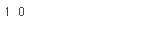Since we are writing more symbols than we are "reading", the sequence never ends.

## The Golden String contains a copy of itself

The sequence contains a copy of itself since we can apply the above process backwards:
• Start by pointing at the left hand end of the (infinite) Fibonacci rabbit sequence with your left hand and with the right get ready to start writing another series.
• If you are pointing at "10", then write down "1".
Otherwise you will be pointing at a "1", so write down a "0".
• Move your left hand past the symbols you have just "read" and repeat the previous step as often as you like.
You will find that your right hand is copying the original sequence, but at something like 0·6 of the speed (actually, at 0·618034... of the speed!!).

####Things to do1. Looking at the other ways of generating the Rabbit sequence above, can you adapt them to
• find another way of writing down the golden string by replacing groups of bits pointed at by your left hand by bits written with your right hand?
• Use your answer "backwards" to find another way in which the golden string contains a complete copy of itself
2. Look at the number of bits read and the number of bits written at each stage. Make a table of these two. What is the ratio between them? Do you notice the Fibonacci numbers appearing? This shows that the ratio of the two (the number of bits used to the number of bits written) will approach phi (0·6180339..).
3. Here is another way to show the Golden sequence contains a copy of itself.
We "read" digits with our left hand again, one at a time, and the right hand will hop over one or two digits, crossing off the next digit. Both hands start at the leftmost digit of the golden sequence. The crossed off digits are still "readable" by the left hand when we come to them, by the way.
If we are pointing at a 1 with the left hand, then hop over TWO digits with the right hand and cross off the next.
If we are pointing at a 0 then hop over ONE digit with the right hand and cross off the next. [In other words, hop over one more digit than you are looking at and cross off the next.]

Here's how the process starts:

```
^ is left-hand-pointer  and v is the right hand pointer
- indicates a digit hopped over by the right hand
X indicates the digit below is to be crossed off by the right hand
+ is a crossed-out 1 and
8 is a crossed-out 0:
Here is the starting position:
v
1 0 1 1 0 1 0 1 1 0 1 1 0 1 0 1 1 0 1 0 1 1 0 ...
^

- - X
1 0 1 1 0 1 0 1 1 0 1 1 0 1 0 1 1 0 1 0 1 1 0 ...
^ Hop over the first two digits and cross off the third

- X
1 0 + 1 0 1 0 1 1 0 1 1 0 1 0 1 1 0 1 0 1 1 0 ...
^   Hop over one and cross off the next

- - X
1 0 + 1 8 1 0 1 1 0 1 1 0 1 0 1 1 0 1 0 1 1 0 ...
^  Hop over two since we are pointing at a (crossed-out) 1

- - X
1 0 + 1 8 1 0 + 1 0 1 1 0 1 0 1 1 0 1 0 1 1 0 ...
^
- X
1 0 + 1 8 1 0 + 1 0 + 1 0 1 0 1 1 0 1 0 1 1 0 ...
^
- - X
1 0 + 1 8 1 0 + 1 0 + 1 8 1 0 1 1 0 1 0 1 1 0 ...
^
- X
1 0 + 1 8 1 0 + 1 0 + 1 8 1 0 + 1 0 1 0 1 1 0 ...
^
- - X
1 0 + 1 8 1 0 + 1 0 + 1 8 1 0 + 1 8 1 0 1 1 0 ...
^
We now have:
1 0 + 1 8 1 0 + 1 0 + 1 8 1 0 + 1 8 1 0 + 1 0 ...
and removing the crossed-off digits gives:
1 0   1   1 0   1 0   1   1 0   1   1 0   1 0 ...
```
which is, of course, the original sequence.
We have shown the golden sequence is self-similar.
• Continue the process above for some more digits of the golden sequence and check it.
• What do you notice about the digits we have removed?

## Fibonacci and the Mandelbrot Set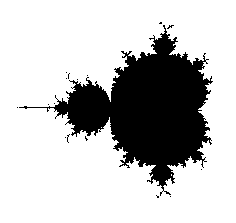The Mandelbrot set shown here has been written about often in maths books, appears in magazines and posters, greeting cards and wrapping paper and in lots of places on the Net.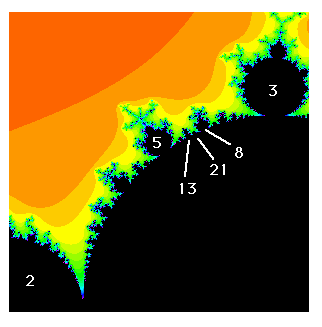A detail from the Mandelbrot set picture is shown here. It is also a link to a page on how the Fibonacci numbers occur in the Mandelbrot Set (at Boston University Mathematics Department).
Mandelbrot Explorer is Panagiotis Christias's Mandelbrot set web page. Click on the image and zoom in and out to explore ever deeper.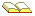The Mandelbrot Set, the Farey Tree and the Fibonacci Sequence R L Delaney, American Mathematical Monthly vol 106 (1999), pages 289-302.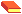M R Schroeder Number Theory in Science and Communication, With Applications in Cryptography, Springer-Verlag, 1990. ISBN 3540158006.Fractals, Chaos and Power Laws by M R Schroeder, 1992, Freeman, ISBN 0 716 72357 3. This is a fascinating book with interesting sections on Phi, the Golden sequence chaos and fractals, and the many places in nature and science where a power law applies (that is, a law of the form y=a xp, where y is proportional to a power of x) although it is somewhat technical.Goedel, Escher, Bach, D Hofstadter, Basic Books, (20th Anniversary Edition, 1999), 800 pages, is a fascinating, funny, intriguing book introducing you to the Escher's amazing pictures, Bach's contrapuntal music and the mathematical patterns in his fugues and how these illustrate Goedel's foundational theorem proved in the 1930's). See page 137.The Fibonacci Tree, Hofstadter and the Golden String K P Togneti, G Winley, T van Ravenstein in Applications of Fibonacci Numbers, 3rd International Conference, (editor: G Bergum), pages 325-334.Characterization of the Set of values f(n)=[n alpha], n=1,2.. by A S Fraenkel, J Levitt, M Shimshoni, in Discrete Mathematics Vol 2, 1972, pages 332-345.© 1996-2008 Dr Ron Knott